## //----------------------------// ArtMatic 1 in 4 out components //----------------------------//

Introduction
Set Constant
Set Vector (Ax,Bx,Cx)
MFD Constant
Color line
RGB Neon line
RGBa half plane
RGB gamma
Shifted Harmonics
Scaled Harmonics
Random color
Hue color
RGBa Sin x
Random Verticals
Unpack
14 Compiled tree

## Introduction

1->4 components are very similar to 1->3 components. There are three primary uses: to map a scalar values (single value streams) to RGBA, to create four independent values from a single value, to unpack a packed RGBA stream, to create four constant values. These components are often especially useful for designing elements for ArtMatic Voyager as they can provide a color texture from elevation map or DFRM object.

In most cases the fourth output either passes out the input value unchanged or provides an alpha channel mask for the RGB image passed out the first three outputs. Those components that pass the input value through the fourth output unchanged are useful for creating trees that will be used by ArtMatic Voyager for both the color map and the elevation map. When passing a surface value into the component, the result is the color shading used to color the surface (from the first three outputs) and the surface elevation map passed out the fourth output. Those components that pass out an alpha mask are especially useful for creating images/animation that will be composited with other images in vQuartz or another image editing program. When used in ArtMatic with the RGB Alpha Bump shader, the result is a texture whose color is provided by the main gradient and whose contours are defined by the texture that feeds the component.

##14 Set Constant

parameters :
A : X/Red Value (0. : 1.)
B : Y/Green Value (0. : 1.)
C : Z/Blue Value (0. : 1.)

discussion :
This component creates a 4D vector constant with the alpha (4th output) either passed trough or set depending on the algorithm.

algorithms :
• Pass & scale input:
Sets a 3 vector constant and pass the alpha with scaling. Scale Alpha (parameter D) scales the input value before it is passed out (the fourth output).
• Set constant alpha:
Sets a 4 vector constant including the alpha.
• Set color & pass alpha
Use this component when you need to add a constant color to a Voyager surface (such as a planet texture or DFRM object). Pass the DFRM object’s output to Set Constant and define the object’s color with slider’s A-C. This component can also be useful in Voyager for creating video transitions where the fourth output is treated as an alpha channel.

##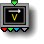14 Set Vector (Ax,Bx,Cx)

parameters :
A : A (-12. : 12.)
B : B (-12. : 12.)
C : C (-12. : 12.)

discussion :
Scales x by vector (A,B,C,1) The input x is passed through the fourth output unchanged. The result is (Ax,Bx,Cx,x).

##14 Add (x+A,x+B,x+C)

parameters :
A : A (-50. : 50.)
B : B (-50. : 50.)
C : C (-50. : 50.)

discussion :
Adds the vector (A,B,C,0) to x and pass x unchanged on fourth output w. The result is (Ax,Bx,Cx, x). This component can be used for colorizing gray shade inputs, creating various offsets for displacements and pure maths applications.

##14 MFD Constant

parameters :
A : Roughness (0. : 1.)
B : Shiningness (0. : 1.)
C : Hilights spread (0. : 1.)
D : Specular level (0. : 1.)

discussion :
This component is useful for creating MFD (MicroFacet Distribution) values that are used by ArtMatic Voyager. This is generally used to feed an ArtMatic extra output that is being used for MFD Shading in Voyager. This component is a true constant. The input is entirely ignored. The difference between MFD Constant and Set Constant is that Set Constant scales the input and sends it out the fourth output; whereas MFD Constant is a true constant that ignores the input value entirely.

##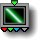14 Color line

parameters :
A : Contrast % (0. : 100.)
B : Width (0. : 8.)
C : Color Ramp (-1. : 1.)

discussion :
coming soon...

##14 RGB Neon line

parameters :
A : Red (0. : 1.)
B : Green (0. : 1.)
C : Blue (0. : 1.)
D : Spread % (0. : 1.)

discussion :
RGB+Alpha implementation of the 1->3 RGB Neon Line. The fourth output is an alpha mask that matches the contours of the RGB output. This version of the component is useful when you want an alpha channel that provides a mask so that only the line (or lines in an iterative system) is visible and not the background. This is very handy when the resulting image or animation will be composited with other images.

##14 RGBa half plane

parameters :
A : Amplitude (-8. : 8.)
B : Offset (-16. : 16.)
C : Color Cycle (0. : 1.)
D : Color Saturation (0. : 1.)

##14 Main Gradient

parameters :
A : Gradient Scale X (0. : 8.)
B : Gradient Offset (-2. : 2.)

discussion :
Map the input value to a color of the current gradient. This component is identical to the
1->3 version of this component except that it passes the input value unchanged through the fourth output.

algorithms :
• Linear:
• Linear mirror:
• Logarithmic:
• Logarithmic mirror:
• Dual Logarithmic:
• Cyclic sin
Cyclic sin uses a sine wave to loop the input. Thus the gradient progresses back and forth indefinitely. 'Scale X' controls the period and 'Offset' the phase. This mode corresponds roughly to the Cyclic clut mode for scalar 1D output system. In 8.08 the entire gradient is looped whatever the period.
• Cyclic mod(ulo)
Cyclic modulo uses a saw tooth wave to loop the input before mapping into the gradient colors. As a result the gradient progresses forwards only while looping so to achieve continuity you'll need that the first color matches the last color. 'Scale X' controls the period and 'Offset' the phase. In 8.08 phases are adjusted so that first instance overlaps the non looping mode.

##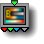14 Indexed Gradient

parameters :
A : Scale X (0. : 8.)
B : Offset (-2. : 2.)
C : Index (0 : 48)

discussion :
This is the same as the 1->3 counterpart with the additional capability that it passes out the input via the alpha channel. This is useful for applying gradient color to a DFRM object or a planet texture. Pass a DFRM object (or planet texture) to this component and the output is RGB color derived from an indexed gradient (selected by parameter C) plus the surface. Great for Voyager RGBA color. Starting engine 8.08 14 Indexed Gradient has the same algotithm options than 13 Main Gradient.

algorithms :
• Linear:
• Linear mirror:
• Logarithmic:
• Logarithmic mirror:
• Dual Logarithmic:
• Cyclic sin:
• Cyclic mod(ulo):

##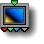14 Shaded Plain Color

parameters :
A : Red (0. : 1.)
B : Green (0. : 1.)
C : Blue (0. : 1.)
D : Contrast % (0. : 100.)

discussion :
RGBA version of the
13 Shaded plain color component. The input value is passed out through the alpha channel.

algorithms :
• Dual color (legacy):
• Single color contrast:
• Dual color contrast:
• Dual Aux color A contrast:
• Dual Aux color B contrast:
• Dual color ramp:
• Triple color ramp :

##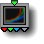14 RGB gamma

parameters :
A : Gamma red (0.12 : 8.)
B : Gamma green (0.12 : 8.)
C : Gamma blue (0.12 : 8.)

discussion :
This is the same as the 1->3 RGB Gamma component with the additional capability that it passes out the input via the alpha channel.

##14 Shifted Harmonics

parameters :
A : Frequency (0. : 12.57)
B : Amplitude (-8. : 8.)
C : Harmonic Offset (0 : 32)

discussion :
This component is mostly used in Sound mode. It can also be used as a color shader. Frequency determines the harmonic pitch. Amplitude determines the pixel brightness/ sound volume. When Harmonic Offset is 0, the output is the reference pitch plus the first three harmonics. When Harmonic Offset is non-zero the same offset is added to the frequency of each harmonic resulting in unusual harmonic relationships (since the harmonics are shifted by a constant frequency rather than a multiplier).

##14 Scaled Harmonics

parameters :
A : Frequency (0. : 12.57)
B : Harmonic Spacing (1. : 6.)
C : Harmonic Offset (-1 : 24)

discussion :
This component is mostly in Sound mode. It can also be used as a color shader. It generates a reference pitch plus three harmonics. When the spacing and offset are set to the minimum values, the result is the reference pitch plus the first three harmonics of the harmonic series. Increasing the spacing, increases the spacing of the harmonics and harmonic offset shifts the harmonics.

##14 Random color

parameters :
A : Color Variance (-2. : 2.)
B : Saturation (0. : 1.)
C : Luminance (0. : 1.)

discussion :
This component can be used as either a color shader that generates continuous random colors or as a mathematical primitive that generates four independent continuous random numbers. If you need four independent random numbers, this component is more efficient than using four 11 random functions.

##14 Hue color

parameters :
A : Hue Variance (0. : 8.)
B : Saturation (0. : 1.)
C : Luminance (0. : 1.)

discussion :
Identical to the 13 component with the addition that it passes the input value out of the fourth output.

##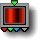14 RGBa Sin x

parameters :
A : Frequency (-4. : 4.)
B : Amplitude (-8. : 8.)
C : Color Cycle (0. : 1.)

discussion :
RGB+Alpha implementation of the sine function. The fourth output is sin x. The input value is mapped to a color of constant hue whose brightness varies with the input value. The color is defined by the color cycle parameter.

##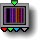14 Random Verticals

parameters :
A : Amount (0. : 1.)
B : Color Cycle (0. : 1.)
C : Frequency (0. : 8.)

discussion :
Color shader that maps input values to randomly regenerated RGB values. The fourth output is an alpha mask of the RGB output. When attached to the global X co-ordinate, the result is colored vertical stripes interspersed with black. The Amount parameter determines the stripe ‘density’ while Frequency determines the width of the stripes. Color Cycle determines the starting color and color range. When Color Cycle is 0, all stripes are black. As the Color Cycle parameter approaches 0, the color saturation approaches 0.

##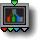14 Unpack

parameters :
No parameters.

discussion :
Unpack an RGB+Alpha stream into its constituent parts. Use this component when you need to access the values being passed in a packed stream.

parameters :
A : Scale 0:1
B : Iterations